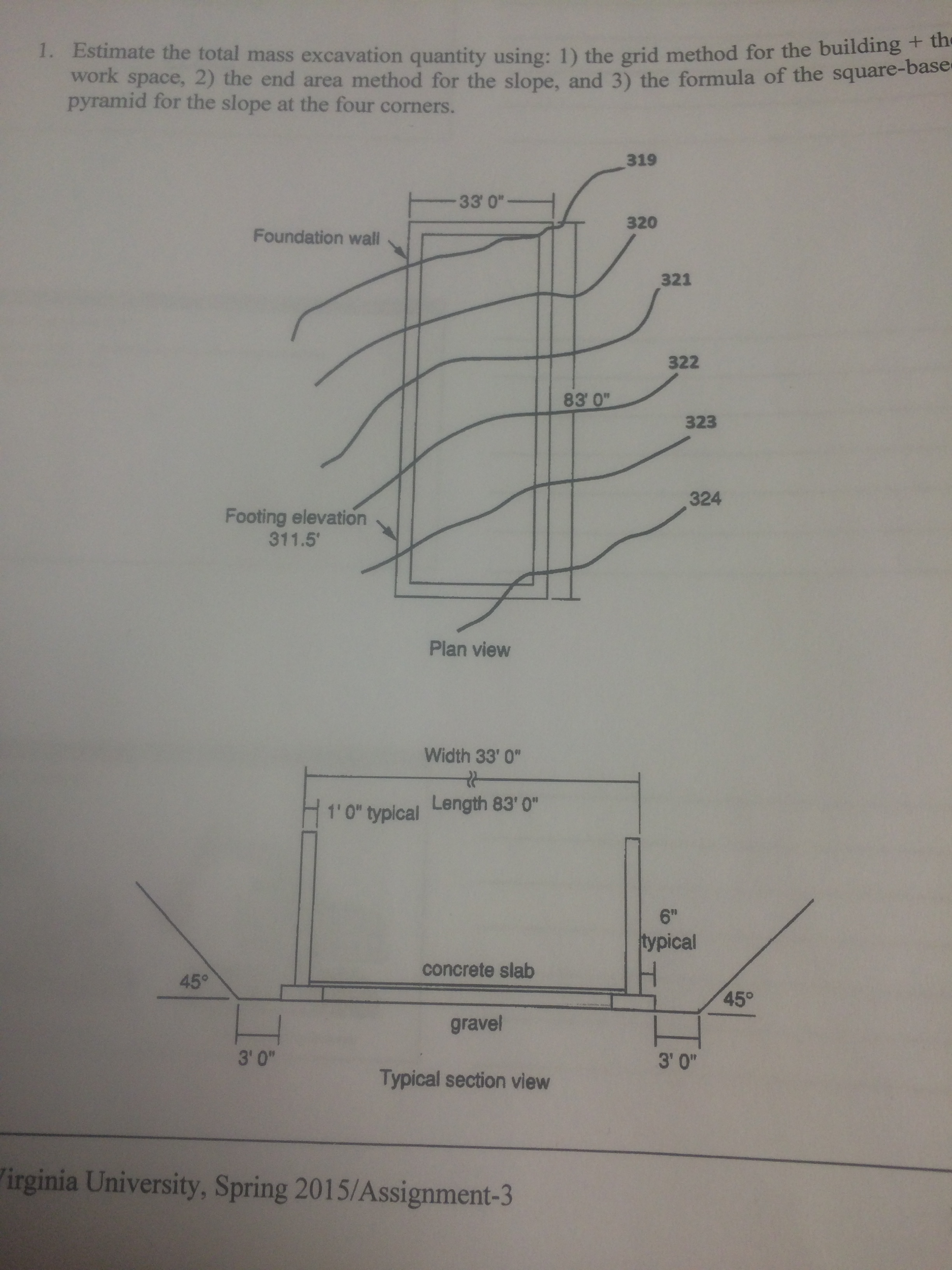### Create an Account

Home / Questions / Estimate the total mass excavation using the grid method for the building the work space,...

# Estimate the total mass excavation using the grid method for the building the work space, 2) the end are method for the slope and 3 the formula of the square-based pyramid for the slope at the

Estimate the total mass excavation using: 1) the grid method for the building + the work space, 2) the end are method for the slope, and 3) the formula of the square-based pyramid for the slope at the four corners.May 26 2020 View more View LessSubscribe To Get Solution